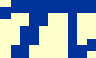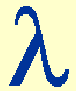1 Show that the plate scale, p, of an astronomical telescope with focal length, F, is given by the relation p = 206265 / F, where p is measured in arcseconds per mm. We have seen that the relationship between the size of an image in the focal plane, s, and its angular size on the sky,, is given by s = F. If F is measured in mm, the platescale in radians per mm is given by p =/ s = 1 / F. There are 180o inradians and hence (180x3600/) arcseconds in 1 radian. Therefore, the platescale in arcseconds per mm is given by p = 206265 / F.

 2 An amateur astronomer has a 20 cm telescope with a focal ratio of f/8. What is the diffraction-limited resolution of this telescope when observing light of wavelength 550nm? Quote your answer both in arcseconds on the sky and mm in the focal plane. Rayleigh's criterion tells us that the diffraction-limited resolution of a telescope,, is given by= 1.22/ D. In the question, the diameter of the telescope aperture, D, is 0.2m. Remembering to use the same units for the wavelength,, this gives= 3.355x10-6 radians. There are (180x3600/) arcseconds in 1 radian, so the diffraction-limited resolution of the amateur astronomer's telescope is 0.69 arcseconds on the sky. To convert resolution from angular units to a linear distance in the focal plane, it is necessary to know the plate scale, p, which in turn requires a knowledge of the telescope focal length, F. The focal length can be determined from the focal ratio, f, as follows: F = Df = 1600mm. The platescale can then be calculated: p = 206265 / F = 129 arcseconds per mm (or "/mm). The diffraction-limited resolution expressed as mm in the focal plane is then simply 0.69/129=0.005mm.

 3 An amateur astronomer has a dark-adapted eye pupil of diameter 8mm and uses a 25 cm telescope with a focal ratio, f/10. What focal length eyepieces are required to operate the telescope at the minimum and maximum useful magnifications? In the question, the diameter of the telescope aperture, D, is 250mm and its focal length, F = Df = 2500mm. A lower limit to the useful magnification of a telescope is given by the expression M ≥ D / d, where d is the diameter of the eye's pupil. Hence M ≥ 31. The magnification is related to the focal length of the eyepiece, by the relation M = F / Fe. Hence an eyepiece of focal length Fe = 80mm would be required. An upper limit to the useful magnification of a telescope is given by the expression M ≤ D, where D is expressed in mm. Hence M ≤ 250, and an eyepiece of focal length Fe = F / M = 10mm would be required.